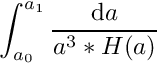driftfac Class Reference

#include <driftfac.h>

## Public Member Functions

void init_drift_table (void)

double get_drift_factor (integertime time0, integertime time1)

double get_gravkick_factor (integertime time0, integertime time1)

double get_hydrokick_factor (integertime time0, integertime time1)

double get_comoving_distance (integertime time0)

double get_comoving_distance_for_scalefactor (double ascale)

double get_scalefactor_for_comoving_distance (double dist)

integertime get_gravkick_factor_inverse (double fac)

## Static Public Member Functions

static double hubble_function (double a)

## Detailed Description

Definition at line 27 of file driftfac.h.

## ◆ get_comoving_distance()

 double get_comoving_distance ( integertime time0 )

Definition at line 191 of file driftfac.cc.

## ◆ get_comoving_distance_for_scalefactor()

 double get_comoving_distance_for_scalefactor ( double ascale )

Definition at line 199 of file driftfac.cc.

## ◆ get_drift_factor()

 double get_drift_factor ( integertime time0, integertime time1 )

This function integrates the cosmological prefactor for a drift step between time0 and time1. The value returned isA lookup-table is used for reasons of speed.

Definition at line 68 of file driftfac.cc.

## ◆ get_gravkick_factor()

 double get_gravkick_factor ( integertime time0, integertime time1 )

Definition at line 109 of file driftfac.cc.

## ◆ get_gravkick_factor_inverse()

 integertime get_gravkick_factor_inverse ( double fac )

Definition at line 220 of file driftfac.cc.

## ◆ get_hydrokick_factor()

 double get_hydrokick_factor ( integertime time0, integertime time1 )

Definition at line 150 of file driftfac.cc.

## ◆ get_scalefactor_for_comoving_distance()

 double get_scalefactor_for_comoving_distance ( double dist )

Definition at line 209 of file driftfac.cc.

## ◆ hubble_function()

 static double hubble_function ( double a )
inlinestatic

Definition at line 39 of file driftfac.h.

## ◆ init_drift_table()

 void init_drift_table ( void )

Definition at line 26 of file driftfac.cc.

The documentation for this class was generated from the following files: